# Civil Engineering - UPSC Civil Service Exam Questions

26.

In the riveted connection shown in the given figure, the rivets subjected to maximum stress would include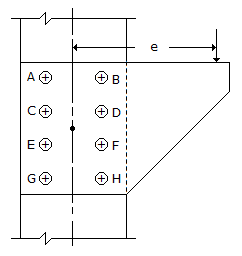A. A, B, C and D B. C, D, E and F C. E, F, G and H D. A, B, G and H

Explanation:

No answer description available for this question. Let us discuss.

27.

An elementary structural frame ABC consists of a vertical member BC and a cantilever beam AB which carries a load P at the free end A, as shown in the given figure. The bending moment diagram consists of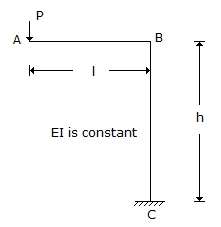A. triangle for both AB and BC B. rectangle for both AB and BC C. triangle for AB and rectangle for BC D. rectangle for AB and triangle for BC

Explanation:

No answer description available for this question. Let us discuss.

28.

Approximate ratio of the permeabilities of two clean soils having D10 = 0.6 mm and D10 = 0.3 mm is

 A. 4 B. 3.75 C. 4.25 D. 3.5

Explanation:

No answer description available for this question. Let us discuss.

29.

The ratio of the torsional moments of resistance of a solid circular shaft of diameter D, and a hollow circular shaft having external diameter D and internal diameter d is given by

 A.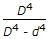B.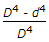C.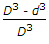D.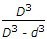Explanation:

No answer description available for this question. Let us discuss.

30.

The force in member AB is in the given figure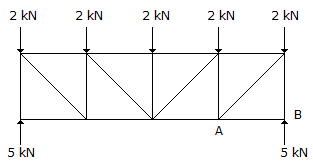A. 5 kN compression B. 2 kN compression C. zero D. 7 kN compression